The Vietnamese Mathematical Hobby Group

MATHEMATICAL TEST PAPER #6

Question 1:

(i) Differentiate with respect to x: (a)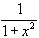(b)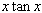(ii) Write down the premitive function of (a)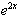(b)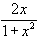(c)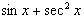(iii) Express sin50.sin15 in the form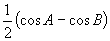.

(iv) If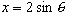and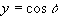, find a relation between x and y which does not involve q.

Question 2:

(i) For what values of the constant B are the graphs of the linear equations 2x + 3y = 6 and 6x + By = 9 are (a) parallel (b) perpendicular ?

(ii) Indicate, using a sketch the region of the Cartesian plane for which 2x + 3y = 6 and 6x + 3y > 9

(iii) Evaluate (a)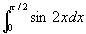(b)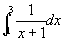Question 3:

(i) Sketch the graph of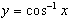.

(ii) (a) Show by completing the square or otherwise, that the expression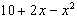can never be greater than 11.

(b) Find the set of values of x for which this expression exceed 7.

(iii) Use the method of math induction to show that the sum of the squares of the first n positive integers is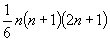.

Question 4:

(i) Show that the tangent to the parabola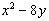at the point (4, 2) intersects the y-axis at (0, -2).

(ii) Transform the parabola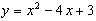to the form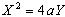and hence find its focal length.

(iii) Sketch the region in the Cartesian (x, y) plane bounded by the parabola, its tangent at (3, 0) and the line x = 2. Find the equation of this tangent and hence calculate the area of this region.

Question 5:

(i) Use Simpson's rule with 3 function values to find an approximate value of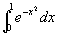.

(ii) For the curve y = f(x) it is given that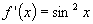and the curve passes through the point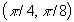. Find the equation of the curve.

Question 6:

(i) (a) Define |x| for a real number x.

(b) Sketch the graph of |x| + |y| = 1.

(ii) (a) Explain the terms "function", "domain" and "range".

(b) Discuss whether the circle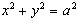in the Cartesian plane is the graph of a function.

(iii) Use one application of Newton's method to estimate that root of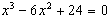which lies near x = 3.

Question 7:

(i) Find the maximum and minimum values of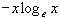for 0 < x < 1.

(ii) Express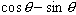in the form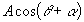in which A is a positive number. Hence or otherwise solve the equation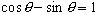for 0 < q < p.

Question 8:

(i) Write down the binomial expansion of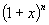and use it to show that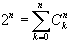.

(ii) (a) For a particle moving in the x-axis with velocity v at time t, show that the acceleration is given by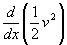.

(b) An object falling directly to the earth from space moves according to the equation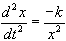, where x is the distance of the object from the centre of the earth at time t. The constant k is related to g, the value of gravity at the earth's surface, and the radius of the earth R, by the equation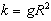. Show that if the object started from rest at a distance of 109 metres from the earth' s centre, then it will reach the earth with a velocity of approximately 11200 m/s. (Assuming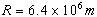, g = 9.8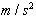)

Question 9:

(i) The first two terms of a GP sequence are 1/2 and 1/8. Find

(a) the n-th term (b) the sum of the first n terms (c) the sum to infinity.

(ii) A and B, in order, continue to toss a coin until a head is thrown, and the first to throw a head wins. Using the result of (i) or otherwise, find the probability that

(a) the game ends at the ninth toss.

(b) the game ends in less than 2n tosses and A wins

(c) A wins.

Question 10:

(i) Calculate the volume of the solid generated when the region in the first quadrant bounded by the curve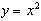, the x-axis and the line y = 4 is rotated about

(a) the y-axis (b) the x-axis.

(ii) Almost always in the application of mathematics to the real world, one has available information which, though interesting, is not really essential for the solution of the problem in hand. The following question contains some of this, so called redundant, information.

As aspirin tablets deteriorate, a quantity of salicyclic acid is formed; to be acceptable, the tablets must not contain salicyclic acid than 0.15 % of the weight of aspirin stated to be in the tablet. From a large supply of tablets, 10 are selected at random and tested. If 9 of more meet the requirement, the lot is passes as satisfactory; otherwise it is rejected. Find the probability of acceptance if, in the supply as a whole, there are 10% unsatisfactory tablets.